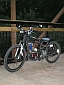# 1 HP Scott motor, Calculations, from the battery side

4 posts / 0 new
echuckj5Offline
Last seen: 11 years 10 months ago
Joined: Wednesday, November 29, 2006 - 07:13
Points: 394
1 HP Scott motor, Calculations, from the battery side

The problem. Motor Heat
Why. according to a chart, The scott motor should not be run at much more than 3000 rpm
The solution. Gear change

Question? finding the efficiency at the 2 gear ratio's, from the battery side

The equation: Watts=VI+I^2R

What do I know?
Battery Voltage: 24 volts
Average speed: 19 mph or 27.86ft/s (from the bicycle computer)
Cruising speed: 25.5 mph
Battery cap: 864 watt/hours
Battery cap: available, 576 watt hours
Distance, 10.4 gearing: 5.7 mi (using 1/2 available cap)
Distance, 9.1 gearing: 7 mi (7.5 on last trip)(using 1/2 available cap)
Watts needed: 750 watts (from kreuttzer's bike calculator)
Watts/hours used: 288 watts (1/2 of avail. cap, battery voltage at 12.2)
VI: 750 watts

Current:
Motor resistance: (the motor is being treated as a resister)
Total watts (10.4):
Total watts (9.1)
Wasted watts(10.4):
Wasted watts (9.1)
Efficiency (at 10.4 ratio)
Efficiency (at 9.1 ratio)

Solve for current:
Watts needed(VI)= Battery Voltage * Current
Watts needed/Battery Voltage = Current
750/24 = 31.25 amps

Solve for Total watts:

echuckj5Offline
Last seen: 11 years 10 months ago
Joined: Wednesday, November 29, 2006 - 07:13
Points: 394
Re: 1 HP Scott motor, Calculations, from the battery side

I need a rate.

I don't know the Voltage Drop across the motor. So, the circuit is, battery, motor, resistor, back to battery. The motor is a resistor in this circuit, it is 750watts. The resistor is the heat loss.

I don't know the average time to go 5.7 miles.
I need a rate. I use 288 watts to travel 5.7 miles. At the 10.4 to 1 ratio.

Lets calculate the time. 5.7 miles/(25.5miles/hr)= .2235 hours

288 watts/.2235 hours = 1288 watts an hour
The motor uses 750 watts an hour.

Total power - mechanical power = wasted power

1288 watts - 750 watts = 538 watts (effect of resistor in circuit)

Total circuit resistance:
538 watts = I^2R
current = 31.25 amps
current squared = 976
watts/squared current = resistance = 538watts/976 = .551 ohm

Now, with very little testing, I calculated 7 miles, and recorded one test 7.5 miles, I have one calculated datum, and one actual datum. It is all I have. Using the 9.1 ratio and 288 watts from the battery pack.

Average distance using the 9.1 ratio and 288 watts: 7.25 miles

Let's calculate the time

7.25 miles/(25.5miles/hour)=.2843 hours

288 watts/.2843 hours = 1013 watts/hour

Total of 1013 watts - 750 watts = 263 watts of wasted energy (the effect of the resistor in the circuit)

Efficiencies:
10.4 to 1: 750/1288 = 58%
9.1 to 1: 750/1013 = 74%
gain in Efficiency: (74-58)/74 = about a 21% increase in effiency

Lets do a check, old mileage, 5.7, new mileage, 7.25 (7.25-5.7)/7.25 = 21%

[b]AGM BATTERIES[/b]

MB-1-EOffline
Last seen: 12 years 3 months ago
Joined: Tuesday, May 8, 2007 - 18:31
Points: 385
Re: 1 HP Scott motor, Calculations, from the battery side

Whew!

I hope you ran your calculator on a separate circuit ...
It must have been putting out some major heat = (resistance). :)

If I study this, I should be able to make some calculations regarding my 560W motor.

Just from a layman's perspective in real world use, I can tell you that I'm getting some better mileage with less heat but if I were to look at it this way I would be ignoring so many factors.

What I can do, now that you showed us how you got to your efficiency rates, is try to garner the same information from my setup to see where I come out. Thanks for sharing your calcs with us Chuck. It gives me some hope that I may someday be able to quantify what is happening in my system and adjust accordingly.

... Thanks ...

Dave B

MB-1-E
<a href="http://visforvoltage.org/book-page/996-mountain-bike-conversion-24v-3-4h... - Bridgestone MB-1 Mountain Bike</a>

echuckj5Offline
Last seen: 11 years 10 months ago
Joined: Wednesday, November 29, 2006 - 07:13
Points: 394
Re: 1 HP Scott motor, Calculations, from the battery side

The correct way to do this, treating the motor as a resistor, measure the voltage drop of the motor and on a second voltmeter, the voltage drop across the controller. then you could solve for the resistance of the controller and the motor. I am just measuring the voltage drop of the "whole" system, which is battery voltage. If voltage drops from the motor and the controller don't add up to the battery voltage, there would be additional resistance, bad connections, too much wire, etc. etc. etc.,, I have never done this, would be done mainly to check the condition of the components and wiring, would give one a baseline for the future.

Not the way to design a vehicle, this is a way to diagnose a vehicle. Thank Andrew, He put the spur in my saddle. I never would have thought to approach it like this.

chuck

Someday, I need to start keeping a log, mercy, mercy,

[b]AGM BATTERIES[/b]

## Who's online

There are currently 0 users online.

## Who's new

• Nickel
• Florix
• gray62
• gregorbrown
• jimtmcdaniels